### Herons Formula - Test Papers

CBSE Test Paper 01

CH-12 Herons Formula

1. The perimeter and area of a triangle whose sides are of lengths 3 cm, 4 cm and 5 cm respectively are
1. 12 cm, 6 $c{m}^{2}$
2. 12 cm, 12 $c{m}^{2}$
3. 6 cm, 6 $c{m}^{2}$
4. 6 cm, 12 $c{m}^{2}$
2. Each equal side of an isosceles triangle is 13 cm and its base is 24 cm Area of the triangle is :
1. $40\sqrt{3}\phantom{\rule{thickmathspace}{0ex}}c{m}^{2}$
2. $25\sqrt{3}\phantom{\rule{thickmathspace}{0ex}}c{m}^{2}$
3. 60 $c{m}^{2}$
4. $50\sqrt{3}\phantom{\rule{thickmathspace}{0ex}}c{m}^{2}$
3. An isosceles right triangle has area 8 $\phantom{\rule{thickmathspace}{0ex}}c{m}^{2}$. The length of its hypotenuse is
1. $\sqrt{32}$ cm
2. $\sqrt{24}$ cm
3. $\sqrt{16}$ cm
4. $\sqrt{48}$ cm
4. If side of a scalene $\mathrm{△}$ is doubled then area would be increased by
1. 200%
2. 25 $\mathrm{%}$
3. 50 $\mathrm{%}$
4. 300 $\mathrm{%}$
5. One of the diagonals of a rhombus is 12cm and area is 96 sq cm. the perimeter of the rhombus is
1. 72 cm
2. 40 cm
6. Fill in the blanks: The area of a triangle of base 35 cm is 420 cm2, then its altitude is ________ cm.
7. Fill in the blanks: The altitude of an equilateral triangle ABC is ________.
8. The base and the corresponding altitude of a parallelogram are 10 cm and 7 cm, respectively. Find its area.
9. How many times area is changed, when sides of a triangle are doubled.
10. Find the area of a triangle whose sides are 9 cm, 12 cm and 15 cm.
11. An isosceles triangle has perimeter 30 cm and each of the equal sides is 12 cm. Find the area of the triangle.
12. The base of a right-angled triangle measures 4 cm and its hypotenuse measures 5 cm. Find the area of the triangle.
13. The perimeter of a triangle is 300 m. If its sides are in the ratio 3 : 5 : 7 . Find the area of the triangle.
14. A kite in the shape of a square with diagonal 32 cm and an isosceles triangle of base 8 cm and side 6 cm each is to be made of three different shades as shown in a figure. How much paper of each shade has been used in it? (Use $\sqrt{5}$ = 2.24)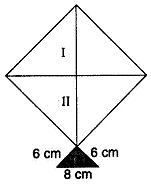15. Two parallel side of a trapezium are 60 cm and 77 cm and other sides are 25 cm and 26 cm. Find the area of the trapezium.

CBSE Test Paper 01
CH-12 Herons Formula

Solution

1. (a) 12 cm, 6 $c{m}^{2}$
Explanation: Perimeter of triangle = 3 + 4 + 5 = 12 cm

Now, s = $\frac{3+4+5}{2}=6$ cm

Area = $\sqrt{s\left(s-a\right)\left(s-b\right)\left(s-c\right)}$

$\sqrt{6\left(6-3\right)\left(6-4\right)\left(6-5\right)}=\sqrt{6×3×2×1}$

= 6 sq cm

2. (c) 60 $c{m}^{2}$

Explanation:

s = $\frac{13+13+24}{2}$= 25 cm

Area of triangle = $\sqrt{s\left(s-a\right)\left(s-b\right)\left(s-c\right)}$

$\sqrt{25\left(25-13\right)\left(25-13\right)\left(25-24\right)}$

$\sqrt{25×12×12×1}$

= 60 sq. cm

3. (a) $\sqrt{32}$ cm
Explanation:

Area of isosceles triangle = $\frac{1}{2}$x Base x Height

Since in an isosceles triangle, Base and Height are equal.

=> 8 = $\frac{1}{2}$x Base x Base

=> Base = Height = 4 cm

Hypotenuse = $\sqrt{{4}^{2}+{4}^{2}}$ = $\sqrt{32}$ cm

4. (d) 300 $\mathrm{%}$
Explanation:

Area of triangle with sides a, b, c (A) = $\sqrt{s\left(s-a\right)\left(s-b\right)\left(s-c\right)}$

New sides are 2a, 2b and 2c

Then ${s}^{\prime }=\frac{2a+2b+2c}{2}=a+b+c$

=>     s' = 2s .............(i)

New area = $\sqrt{{s}^{\prime }\left({s}^{\prime }-2a\right)\left({s}^{\prime }-2b\right)\left({s}^{\prime }-2c\right)}$

$\sqrt{2s\left(2s-2a\right)\left(2s-2b\right)\left(2s-2c\right)}$

$4\sqrt{s\left(s-a\right)\left(s-b\right)\left(s-c\right)}$

= 4A

Increased area = 4A - A = 3A

% of increased area = $\frac{3\mathrm{A}}{\mathrm{A}}×100$ = 300%

5. (c) 40 cm
Explanation:

d2 = $\frac{\mathrm{A}\mathrm{r}\mathrm{e}\mathrm{a}×2}{{d}_{1}}$

$\frac{96×2}{12}$

=16 cm

length of side of rhombus = $\sqrt{{6}^{2}+{8}^{2}}$ = 10 cm

perimeter of rhombus = 4 x side

= 4  x 10 = 40 cm

6. 24

7. $\frac{\sqrt{3}}{2}a$

8. The base of parallelogram =10 cm  and the corresponding altitude = 7 cm.
Area of parallelogram = Base $×$ Corresponding altitude
= 10 $×$ 7 = 70 cm2.

9. Area of triangle = $\frac{1}{2}$  × base  × height = $\frac{1}{2}$ × b  × h
If new base B = 2b and height  H = 2h
New area of triangle   = $\frac{1}{2}$ × B  × H   = $\frac{1}{2}$ × 2b  × 2h =4( $\frac{1}{2}$ × b  × h) = 4 (area of triangle)
So doubling the sides leads to 4 times the area.

10. Let a = 9 cm, b = 12 cm and c = 15 cm
Since, 2s = a + b + c
$⇒$ s = $\frac{1}{2}$(a + b + c)
$\frac{1}{2}$(9 + 12 + 15)
$\frac{1}{2}$(36) = 18 cm
Now, area of triangle = $\sqrt{s\left(s-a\right)\left(s-b\right)\left(s-c\right)}$
$\sqrt{18\left(18-9\right)\left(18-12\right)\left(18-15\right)}$
$\sqrt{18×9×6×3}$
= 54 cm2

11.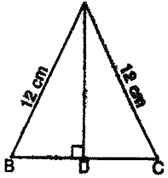a = 12 cm, b = 12 cm
Perimeter = 30 cm
a + b + c = 30
⇒ 12 + 12 + c = 30
⇒ 24 + c = 30
⇒ c = 30 – 24
⇒ c = 6 cm
s = $\frac{30}{2}$ cm = 15 cm
∴ Area of the triangle $=\sqrt{s\left(s-a\right)\left(s-b\right)\left(s-c\right)}$
$=\sqrt{15\left(15-12\right)\left(15-12\right)\left(15-6\right)}$
$=\sqrt{15\left(3\right)\left(3\right)\left(9\right)}=9\sqrt{15}$ cm2

12. Given: base of a right-angled triangle = 4 cm and hypotenuse =  5 cm.
In right-angled triangle ABC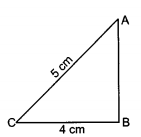AB+ BC2 = AC2 (By Pythagoras Theorem)
$⇒$ AB2 + 42 = 52
$⇒$ AB= 25 - 16 = 9
$⇒$ AB = 3 cm
$\therefore$ Area of $\mathrm{\Delta }ABC=\frac{1}{2}BC×AB=\frac{1}{2}×4×3=6{\mathrm{c}\mathrm{m}}^{2}$
Hence area of given right-angled  triangle is 6 cm2.

13.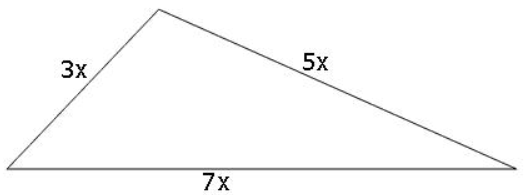Suppose that the sides in metres are 3x, 5x and 7x.
Then, we know that 3x + 5x + 7x = 300 (Perimeter of the triangle)
Therefore, 15x = 300, which gives x = 20.
So the sides of the triangles are 3 $×$ 20 m, 5 $×$ 20 m and 7 $×$ 20 m
i.e., 60m, 100m and 140m.
We have s = $\frac{60+100+140}{2}$ = 150 m
and area will be = $\sqrt{150\left(150-60\right)\left(150-100\right)\left(150-140\right)}$
$\sqrt{150×90×50×10}$
= 1500$\sqrt{3}$ m2

14. Here ABCD be the square and $\mathrm{△}$CEF be an isosceles triangle.
Let the diagonals bisect each other at O.
Then,
AO =$\frac{1}{2}×$ 32 cm
= 16 cm
Area of shaded portion I =
= 256 sq cm
Similarly, Area of shaded portion II =
= 256 sq cm
And, for triangle
base, a = 8 cm
and side, b = 6 cm
Area of portion III =
$=\frac{8}{4}\sqrt{4×\left(6{\right)}^{2}-64}$
$=2\sqrt{144-64}$
$=8\sqrt{5}$
= 17.92 sq cm
Thus, the papers of three shades required are 256 sq cm, 256 sq cm and 17.92 sq cm.

15.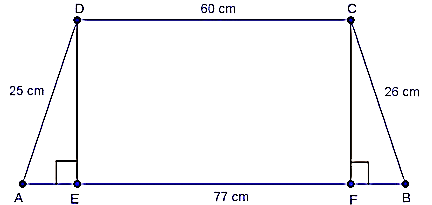Given that,
AB = 77 cm, CD = 60 cm, BC = 26 cm and AD = 25 cm
Now, DE $\perp$ AB and CF $\perp$ AB is drawn.
$\therefore$ EF = DC = 60 cm
Let AE = x
$⇒$ BF = 77 - 60 - x = (17 - x)
In $\mathrm{△}$ADE, DE2 = AD2 - AE2 [pythagoras theorem]
= 252 - x2
And